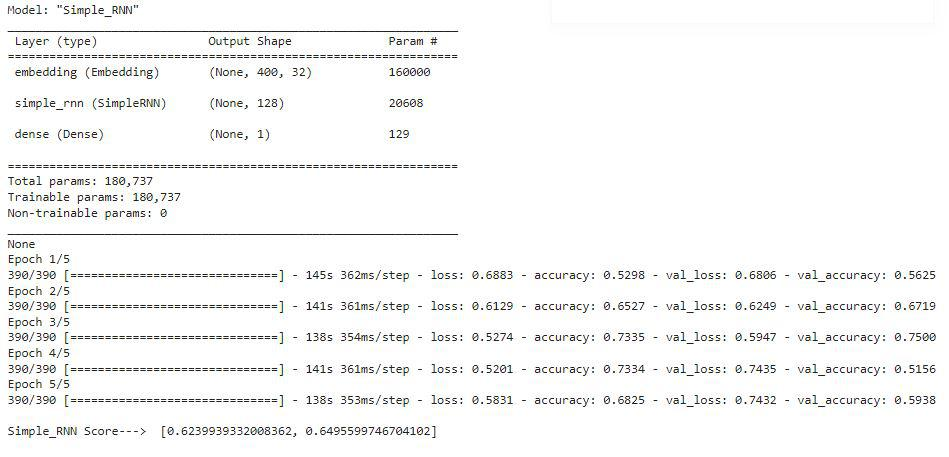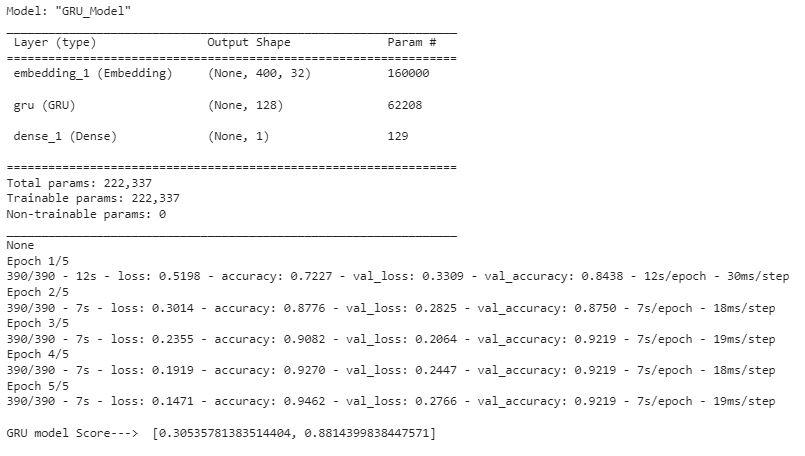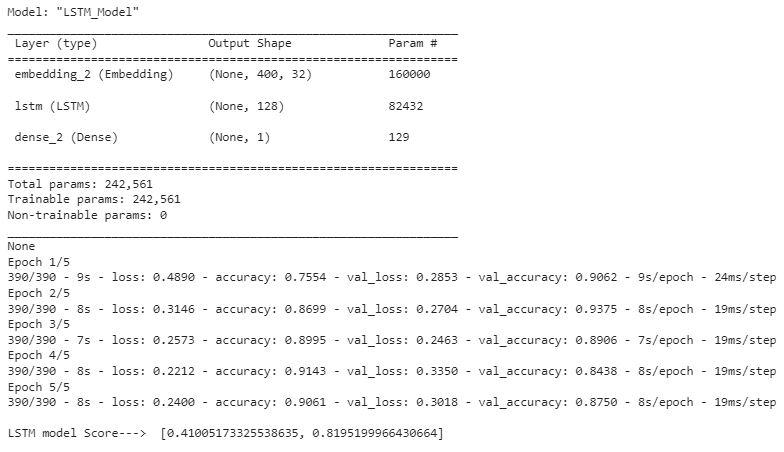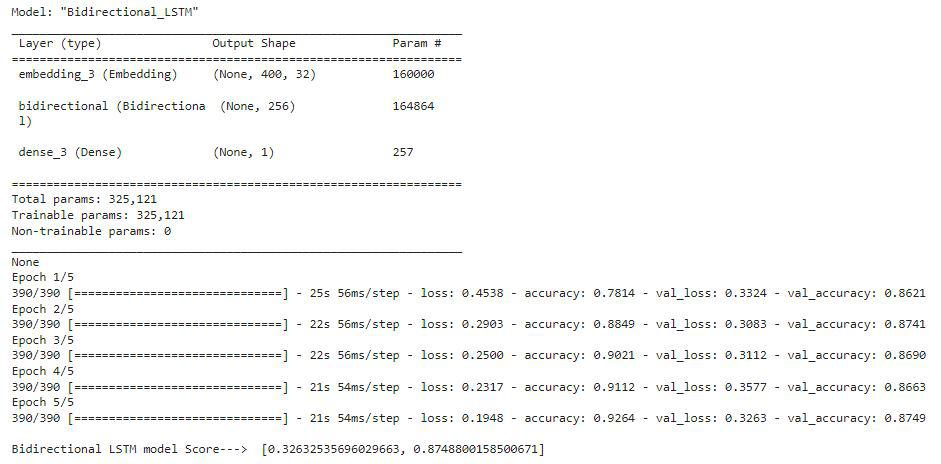Open in App
Not now

# Sentiment Analysis with an Recurrent Neural Networks (RNN)

• Last Updated : 14 Oct, 2022

Recurrent Neural Networks (RNN) are to the rescue when the sequence of information is needed to be captured (another use case may include Time Series, next word prediction, etc.). Due to its internal memory factor, it remembers past sequences along with current input which makes it capable to capture context rather than just individual words. For better understanding, please read the article Introduction to Recurrent Neural Network and related articles in GeeksforGeeks

We will conduct Sentiment Analysis to understand text classification using Tensorflow!

## Python3

 `from` `tensorflow.keras.layers ``import` `SimpleRNN, LSTM, GRU, Bidirectional, Dense, Embedding``from` `tensorflow.keras.datasets ``import` `imdb``from` `tensorflow.keras.models ``import` `Sequential``import` `numpy as np`

We will be using Keras IMDB dataset. vocabulary size is a parameter that is used the get data containing the given number of most occurring words in the entire corpus of textual data.

## Python3

 `# Getting reviews with words that come under 5000``# most occurring words in the entire``# corpus of textual review data``vocab_size ``=` `5000``(x_train, y_train), (x_test, y_test) ``=` `imdb.load_data(num_words``=``vocab_size)` `print``(x_train[``0``])`

Output:

```[1, 14, 22, 16, 43, 530, 973, 1622, 1385, 65, 458, 4468, 66,3941, 4, 173, 36, 256, 5, 25, 100, 43, 838, 112, 50, 670, 2, 9, 35, 480, 284, 5, 150, 4, 172,
112, 167, 2, 336, 385, 39, 4, 172, 4536, 1111, 17, 546, 38, 13, 447, 4, 192, 50, 16, 6, 147, 2025, 19, 14, 22,
4, 1920, 4613, 469, 4, 22, 71, 87, 12, 16, 43, 530, 38, 76, 15, 13, 1247, 4, 22, 17, 515, 17, 12, 16, 626, 18,
2, 5, 62, 386, 12, 8, 316, 8, 106, 5, 4, 2223, 2, 16, 480, 66, 3785, 33, 4, 130, 12, 16, 38, 619, 5, 25, 124,
..]    ```

These are the index values of the words and hence we done see any reviews

## Python3

 `# Getting all the words from word_index dictionary``word_idx ``=` `imdb.get_word_index()` `# Originally the index number of a value and not a key,``# hence converting the index as key and the words as values``word_idx ``=` `{i: word ``for` `word, i ``in` `word_idx.items()}` `# again printing the review``print``([word_idx[i] ``for` `i ``in` `x_train[``0``]])`

Output:

`['the', 'as', 'you', 'with', 'out', 'themselves', 'powerful', 'lets', 'loves', 'their', 'becomes', 'reaching', 'had', 'journalist', 'of', 'lot', 'from', 'anyone', 'to', 'have', 'after', 'out', 'atmosphere', 'never', 'more', 'room', 'and', 'it', 'so', 'heart', 'shows', 'to', 'years', 'of', 'every', 'never', 'going', 'and', 'help', 'moments', 'or', 'of', 'every', 'chest', 'visual', 'movie', 'except', 'her', 'was', 'several', 'of', 'enough', 'more', 'with', 'is', 'now', 'current', 'film', 'as', 'you', 'of', 'mine', 'potentially', 'unfortunately', 'of', 'you', 'than', 'him', 'that', 'with', 'out', 'themselves', 'her', 'get', 'for', 'was', 'camp', 'of', 'you', 'movie', 'sometimes', 'movie', 'that', 'with', 'scary', 'but', 'and', 'to', 'story', 'wonderful', 'that', 'in', 'seeing', 'in', 'character', 'to', 'of', '70s', 'and', 'with', 'heart', 'had', 'shadows', 'they', 'of', 'here', 'that', 'with', 'her', 'serious', 'to', 'have', 'does', 'when', 'from', 'why', 'what', 'have', 'critics', 'they', 'is', 'you', 'that', "isn't", 'one', 'will', 'very', 'to', 'as', 'itself', 'with', 'other', 'and', 'in', 'of', 'seen', 'over', 'and', 'for', 'anyone', 'of', 'and', 'br', "show's", 'to', 'whether', 'from', 'than', 'out', 'themselves', 'history', 'he', 'name', 'half', 'some', 'br', 'of', 'and', 'odd', 'was', 'two', 'most', 'of', 'mean', 'for', '1', 'any', 'an', 'boat', 'she', 'he', 'should', 'is', 'thought', 'and', 'but', 'of', 'script', 'you', 'not', 'while', 'history', 'he', 'heart', 'to', 'real', 'at', 'and', 'but', 'when', 'from', 'one', 'bit', 'then', 'have', 'two', 'of', 'script', 'their', 'with', 'her', 'nobody', 'most', 'that', 'with', "wasn't", 'to', 'with', 'armed', 'acting', 'watch', 'an', 'for', 'with', 'and', 'film', 'want', 'an']`

Let’s check the range of the reviews we have in this dataset.

## Python3

 `# Get the minimum and the maximum length of reviews``print``(``"Max length of a review:: "``, ``len``(``max``((x_train``+``x_test), key``=``len``)))``print``(``"Min length of a review:: "``, ``len``(``min``((x_train``+``x_test), key``=``len``)))`

Output:

```Max length of a review::  2697
Min length of a review::  70```

We see that the longest review available is 2697 words and the shortest one is 70. While working with Neural Networks, it is important to make all the inputs in a fixed size. To achieve this objective we will pad the review sentences.

## Python3

 `from` `tensorflow.keras.preprocessing ``import` `sequence` `# Keeping a fixed length of all reviews to max 400 words``max_words ``=` `400` `x_train ``=` `sequence.pad_sequences(x_train, maxlen``=``max_words)``x_test ``=` `sequence.pad_sequences(x_test, maxlen``=``max_words)` `x_valid, y_valid ``=` `x_train[:``64``], y_train[:``64``]``x_train_, y_train_ ``=` `x_train[``64``:], y_train[``64``:]`

### SimpleRNN (also called Vanilla RNN)

They are the most basic form of Recurrent Neural Networks that tries to memorize sequential information. However, they have the native problems of Exploding and Vanishing gradients. For a detailed understanding of how RNNs works and its limitations please read the article Recurrent Neural Networks Explanation

## Python3

 `# fixing every word's embedding size to be 32``embd_len ``=` `32` `# Creating a RNN model``RNN_model ``=` `Sequential(name``=``"Simple_RNN"``)``RNN_model.add(Embedding(vocab_size,``                        ``embd_len,``                        ``input_length``=``max_words))` `# In case of a stacked(more than one layer of RNN)``# use return_sequences=True``RNN_model.add(SimpleRNN(``128``,``                        ``activation``=``'tanh'``,``                        ``return_sequences``=``False``))``RNN_model.add(Dense(``1``, activation``=``'sigmoid'``))` `# printing model summary``print``(RNN_model.summary())` `# Compiling model``RNN_model.``compile``(``    ``loss``=``"binary_crossentropy"``,``    ``optimizer``=``'adam'``,``    ``metrics``=``[``'accuracy'``]``)` `# Training the model``history ``=` `RNN_model.fit(x_train_, y_train_,``                        ``batch_size``=``64``,``                        ``epochs``=``5``,``                        ``verbose``=``1``,``                        ``validation_data``=``(x_valid, y_valid))` `# Printing model score on test data``print``()``print``(``"Simple_RNN Score---> "``, RNN_model.evaluate(x_test, y_test, verbose``=``0``))`

Output:The vanilla form of RNN gave us a Test Accuracy of 64.95%. Limitations of Simple RNN are it is unable to handle long sentences well because of its vanishing gradient problems.

### Gated Recurrent Units (GRU)

GRUs are lesser know but equally robust algorithms to solve the limitations of simple RNNs. Please read the article Gated Recurrent Unit Networks for a better understanding of their work.

## Python3

 `# Defining GRU model``gru_model ``=` `Sequential(name``=``"GRU_Model"``)``gru_model.add(Embedding(vocab_size,``                        ``embd_len,``                        ``input_length``=``max_words))``gru_model.add(GRU(``128``,``                  ``activation``=``'tanh'``,``                  ``return_sequences``=``False``))``gru_model.add(Dense(``1``, activation``=``'sigmoid'``))` `# Printing the Summary``print``(gru_model.summary())` `# Compiling the model``gru_model.``compile``(``    ``loss``=``"binary_crossentropy"``,``    ``optimizer``=``'adam'``,``    ``metrics``=``[``'accuracy'``]``)` `# Training the GRU model``history2 ``=` `gru_model.fit(x_train_, y_train_,``                         ``batch_size``=``64``,``                         ``epochs``=``5``,``                         ``verbose``=``1``,``                         ``validation_data``=``(x_valid, y_valid))` `# Printing model score on test data``print``()``print``(``"GRU model Score---> "``, gru_model.evaluate(x_test, y_test, verbose``=``0``))`

Output:Test Accuracy of GRU was found to be 88.14%. GRU is a form of RNN that are better than simple RNN and are often faster than LSTM due to its relatively fewer training parameters.

### Long Short Term Memory (LSTM)

LSTM is better in terms of capturing the memory of sequential information better than simple RNNs. To understand the theoretical aspects of LSTM please visit the article Long Short Term Memory Networks Explanation. Due to increased complexity than that of GRU, it is slower to train but in general, LSTMs give better accuracy than GRUs.

## Python3

 `# Defining LSTM model``lstm_model ``=` `Sequential(name``=``"LSTM_Model"``)``lstm_model.add(Embedding(vocab_size,``                         ``embd_len,``                         ``input_length``=``max_words))``lstm_model.add(LSTM(``128``,``                    ``activation``=``'relu'``,``                    ``return_sequences``=``False``))``lstm_model.add(Dense(``1``, activation``=``'sigmoid'``))` `# Printing Model Summary``print``(lstm_model.summary())` `# Compiling the model``lstm_model.``compile``(``    ``loss``=``"binary_crossentropy"``,``    ``optimizer``=``'adam'``,``    ``metrics``=``[``'accuracy'``]``)` `# Training the model``history3 ``=` `lstm_model.fit(x_train_, y_train_,``                          ``batch_size``=``64``,``                          ``epochs``=``5``,``                          ``verbose``=``2``,``                          ``validation_data``=``(x_valid, y_valid))` `# Displaying the model accuracy on test data``print``()``print``(``"LSTM model Score---> "``, lstm_model.evaluate(x_test, y_test, verbose``=``0``))`

Output:LSTM model Provided a test accuracy of 81.95%

### Bi-directional LSTM Model

Bidirectional LSTMS are a derivative of traditional LSTMS. Here, two LSTMs are used to capture both the forward and backward sequences of the input. This helps in capturing the context better than normal LSTM. For more information on Bidirectional LSTM please read the article Emotion Detection using Bidirectional LSTM.

## Python3

 `# Defining Bidirectional LSTM model``bi_lstm_model ``=` `Sequential(name``=``"Bidirectional_LSTM"``)``bi_lstm_model.add(Embedding(vocab_size,``                            ``embd_len,``                            ``input_length``=``max_words))``bi_lstm_model.add(Bidirectional(LSTM(``128``,``                                     ``activation``=``'tanh'``,``                                     ``return_sequences``=``False``)))``bi_lstm_model.add(Dense(``1``, activation``=``'sigmoid'``))` `# Printing model summary``print``(bi_lstm_model.summary())` `# Compiling model summary``bi_lstm_model.``compile``(``  ``loss``=``"binary_crossentropy"``,``  ``optimizer``=``'adam'``,``  ``metrics``=``[``'accuracy'``]``)` `# Training the model``history4 ``=` `bi_lstm_model.fit(x_train_, y_train_,``                             ``batch_size``=``64``,``                             ``epochs``=``5``,``                             ``verbose``=``2``,``                             ``validation_data``=``(x_test, y_test))` `# Printing model score on test data``print``()``print``(``"Bidirectional LSTM model Score---> "``,``      ``bi_lstm_model.evaluate(x_test, y_test, verbose``=``0``))`

Output:Bidirectional LSTM gave a test score of 87.48%.

### Conclusion

• All the major flavors for Recurrent Neural Networks were tested in their base forms keeping all the common hyperparameters like number of layers, activation function, batch size, and epochs to be the same across all the above models. The model complexity increases as we go from SimpleRNN to Bidirectional LSTM as the number of trainable parameters goes up.
• Out of all the models, for the given dataset of IMDB reviews, the GRU model gave the best result in terms of accuracy.

My Personal Notes arrow_drop_up